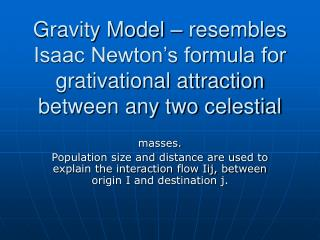DownloadDownload PresentationGravity Model – resembles Isaac Newton’s formula for grativational attraction between any two celestial

# Gravity Model – resembles Isaac Newton’s formula for grativational attraction between any two celestial

Télécharger la présentation## Gravity Model – resembles Isaac Newton’s formula for grativational attraction between any two celestial

- - - - - - - - - - - - - - - - - - - - - - - - - - - E N D - - - - - - - - - - - - - - - - - - - - - - - - - - -
##### Presentation Transcript

1. Gravity Model – resembles Isaac Newton’s formula for grativational attraction between any two celestial masses. Population size and distance are used to explain the interaction flow Iij, between origin I and destination j.

2. Gravity model allows both size and distance to vary simultaneously: • Iij=k PiPj • B • dij • Where Iij=predicted interaction between origin I and destination j. • K=a scaling constant • Pi=a measure of size, usually population for origin i. • Pj=a measure of size, usually population for destination j. • B= am exponent which adjusts for the rate of distance decay unique to the type of interaction being measured.

3. This model can be modified to model all types of spatial interaction. • The mass or size variables in the numerator of the fraction will have a positive relationship with spatial interaction. Thus, as population of a state increases, both for origins and destinations, the interaction between them increases. Distance, being denominator, will be negatively, or inversely related to interaction. Interaction decreases as distance increases. • The other two factors are constants calibrated statistically to produce the most realistic levels of interaction between places.